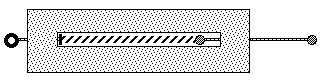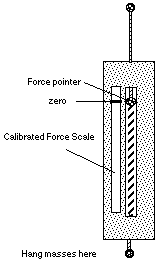# Hands-On-Physics MECHANICS

## - Force -

Building the Thruster
The thruster is both an instrument for measuring force and a tool for applying force in a controlled way. The working part of this thruster is a simple coil spring. Its frame is constructed from cardboard, and the plunger is a bamboo skewer with beads glued on. Pulling the ring at the end of the plunger compresses the springs.Figure M4
Thruster

The frame may be cut from several pieces of corrugated cardboard. The dimensions shown in the plans accommodate the springs in your kit. The lines of corrugation should run the long way to ensure rigidity. For more details about building a Thruster, look at the Thruster Plans which have dimensioned drawings.

Thruster Calibration
The Thruster is a tool for measuring unknown forces and applying known forces. All the Thrusters will be different, so each one must be calibrated; we must agree on a standard.Figure M5
Thruster Calibration

For this calibration we will use the pull of earth's gravity on some mass we agree upon. Physicist use kilograms and grams of masses. Your class could use pennies or nickels for mass instead of metric masses. ( One penny is about 2.5 grams and one nickel is about 5 grams. )
For force, physicists use Newtons. The earth's pull on one gram is about 0.01 Newtons so the pull on 100 grams is about 1 Newton (0.980 N). The Thruster is designed for a maximum force of about 1.5 Newtons, so a 50 g, and 100 g mass would let you determine the spring compression for 0.5 N, 1.0 N, and 1.5 N respectively. Use one edge of the spring-stop bead for a force pointer, mark a zero reference point on the frame beside the spring slot. You could glue a clean white strip of paper beside the spring slot for the force markings. Pull the plunger down, compressing the spring, with a 50 g mass, and mark the location of the force pointer on the frame beside the spring slot. Repeat this with 100 g, and 150 g. Label these marks zero, 0.5 N, 1.0 N, and 1.5 N. Divide up these 0.5 Newton marks, by eye, with five subdivisions (one subdivision = 0.1 Newtons) and you are ready to measure an unknown force or push with a known force.

Thruster Energy
This is an investigation to establish a mathematical relation between the compression of the Thruster spring and the distance it can shoot a puck. You need a calibrated thruster and a puck. One way to build a puck is to glue three 6 cm diameter circles of corrugated cardboard. You could also use a jar cap. A long, smooth, flat surface is necessary; if everyone in class uses the same surface, you can have a contest. The main part of this investigation is the collection of a set of measurement pairs, maximum thruster forces and sliding distances. You could measure slide distances for maximum forces of 0.25 N, 0.50 N, 1.00 N, 1.25 N, and 1.50 N. Record your measurements in a table and plot them on a graph. This all sounds easy, but you may discover that the slide distance is not always consistent. You may need to make several shots at each maximum force and average the slide distance. Record all your measurements, but list and graph only the averages. When you graph recall that the maximum force is the variable which you choose, so it is the independent variable and would normally be plotted horizontally. The slide distances would then be plotted vertically as the dependent variable. Can you express this relationship mathematically?

• If it is a straight line then the slide distance (d) is directly proportional to the force (F).
• Expressed as the equation for a straight line, d = slope*F .
• If it looks like a parabola, square one of the variables and replot to get a straight line.
As the spring of the Thruster is compressed, it stores energy. This energy can be released to move a puck. As the puck slides it loses energy in direct proportion to the distance it slides, giving up energy as it slows down.. If a puck slides twice as far it must have started with twice as much energy.

How does the spring energy change when it is compressed twice as much? (Is energy proportional to compression?)

### Other Messings

• Design and build a puck that slides further.